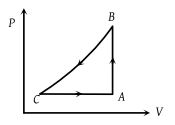# NEET Physics Thermodynamics Questions Solved

A sample of an ideal gas is taken through a cycle a shown in figure. It absorbs 50J of energy during the process AB, no heat during BC, rejects 70J during CA. 40J of work is done on the gas during BC. Internal energy of gas at A is 1500J, the internal energy at C would be(1) 1590 J

(2) 1620 J

(3) 1540 J

(4) 1570 J

(1) $\Delta {W}_{AB}=0$ as V = constant

$\Delta {Q}_{AB}=\Delta {U}_{AB}=50J$ (Given)

${U}_{A}=1500J$${U}_{B}=\left(1500+50\right)J=1550J$

$\Delta {W}_{BC}=-\Delta {U}_{BC}=-40J$ (Given)

$\Delta {U}_{BC}=40J$

${U}_{C}=\left(1550+40\right)J=1590J$

Difficulty Level:

• 36%
• 33%
• 19%
• 14%
Crack NEET with Online Course - Free Trial (Offer Valid Till August 28, 2019)